Strangely Attracted to Chaos

Fractal Images

 Some of these images have a very long history and date back to my first programs to produce fractals, which were written about fifteen years ago. They ran on an IBM PC XT clone. I remember a program running for two days just to produce a two-color [black and orange] image of the Mandelbrot set [like image 08 below] on the 200 x 320 pixel CGA screen. My programs have evolved a lot since then and with the faster computers we have today they can produce images with much higher resolution. For this show there are two image sizes. The 16" x 20" ones are 720 pixels/inch, i.e., 11,520 x 14,400 pixels. The 42.5" x 42.5" ones are 360 pixels/inch, i.e., 15,300 x 15,300 pixels. And some of these took a long time to calculate as well. Several ran for more than four days each. Lyapunov image 11, for instance, ran for 349255 seconds [4 days, 1 hour and 55 seconds] and did a total of 11520 x 14400 x 4000 = 663,552,000,000 iterations [that's 663.5 billion iterations]. Through the years I've read many articles in Scientific American and other science and computing magazines and learned the equations and algorithms to calculate these fractals. I must admit I've had just as much fun writing the programs and getting them to work as I have had seeing the fantastic images that the programs can produce and figuring out how to colour them for best effect. These days you don't have to write your own programs to produce fractal images. The internet has many run-on-the-page and downloadable programs to choose from if you decide to explore fractals on your own. Try running some from the Generators page.

Mandelbrot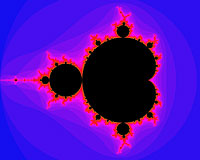Fractal No. 8 (Mandelbrot)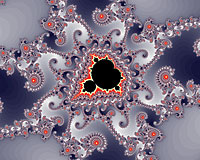Fractal No. 1 (Mandelbrot)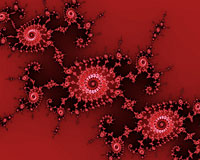Fractal No. 19 (Mandelbrot)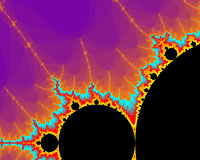Fractal No. 12 (Mandelbrot)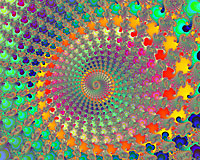Fractal No. 18 (Mandelbrot)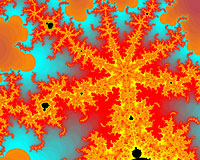Fractal No. 14 (Mandelbrot)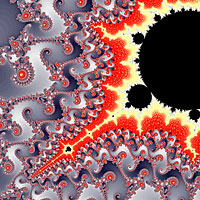Fractal No. 20 (Mandelbrot)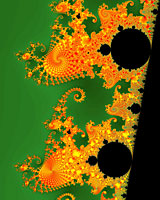Fractal No. 16 (Mandelbrot)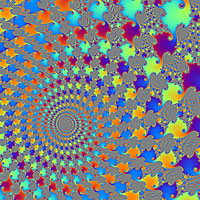Fractal No. 21 (Mandelbrot)

Julia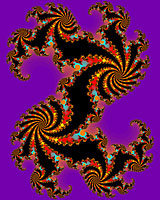Fractal No. 15a (Julia)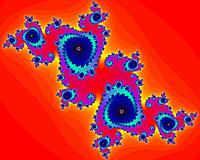Fractal No. 2 (Julia)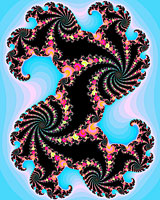Fractal No. 15b (Julia)

Lyapunov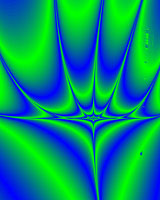Fractal No. 11 (Lyapunov)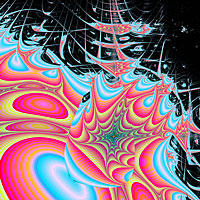Fractal No. 22 (Lyapunov)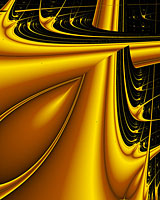Fractal No. 4 (Lyapunov)

Lambda Mode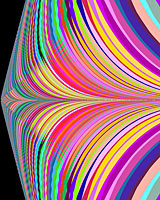Fractal No. 10 (Lambda)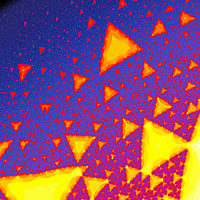Fractal No. 23 (Lambda)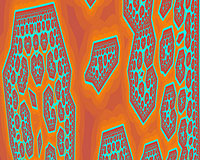Fractal No. 9 (Lambda)

Verhulst Population Model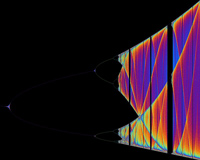Fractal No. 13 (Verhulst)

Model of Magnetism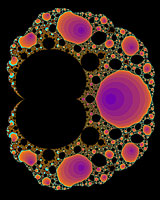Fractal No. 7 (Magnetism)

 Navigation: Strangely Attracted to Chaos...Fractal Images Tour: Previous - Iterators and the Chaos Game   Next - Generators